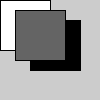# pop_matrix()#

Pops the current transformation matrix off the matrix stack.

## Examples#def setup():
py5.fill(255)
py5.rect(0, 0, 50, 50)  # white rectangle

py5.push_matrix()
py5.translate(30, 20)
py5.fill(0)
py5.rect(0, 0, 50, 50)  # black rectangle
py5.pop_matrix()

py5.fill(100)
py5.rect(15, 10, 50, 50)  # gray rectangle


## Description#

Pops the current transformation matrix off the matrix stack. Understanding pushing and popping requires understanding the concept of a matrix stack. The push_matrix() function saves the current coordinate system to the stack and pop_matrix() restores the prior coordinate system. push_matrix() and pop_matrix() are used in conjuction with the other transformation functions and may be embedded to control the scope of the transformations.

Underlying Processing method: popMatrix

## Signatures#

pop_matrix() -> None


Updated on March 06, 2023 02:49:26am UTC